Tableau

# Predictions with Alteryx

In this post I will show how to leverage the predictive capabilities of Alteryx. The predictive tools available in Alteryx are accessible as a free downloadable package at //downloads.alteryx.com/.The package contains a wide series of R based macros that easily can be implemented in an Alteryx workflow.

These tools differs from the usual workings of Alteryx. Normally the tools used in a workflow are working through the Alteryx engine, but with the predictive tools an R engine is being queried.

For those unfamiliar with R, R is a programming language used for a variety of computing task where statistic and modelling are amongst the capabilities.

The model

In this post I will go through a very simple example of making a predictive model in Alteryx.

Basically this post will be concerned with making a model that can predict the gender of an individual based on height and weight.

When developing such a model our first concern should be the characteristics of what we are trying to predict. In this case what we are trying to predict, the dependent variable, is binary. This simply means that the variable only take on two values, male or female.

Having a binary dependent variable influences our choice of model. In this example we will be using a logistic regression model. We could have chosen a variety of models concerned with binary responses, the logistic model simply being the usual go to model in such matters.The data

We will develop the model based on a dataset containing 10.000 observations and 3 variables or fields. The dataset is of the cross-sectional type, meaning that we have observations on an individual level and no information about time. The 3 fields of the data contains information about gender, height and weight.

The dataset will be available for download at the bottom of the post.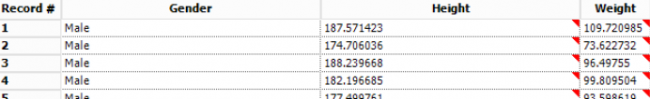Designing the model

For simplifications we will go against usual econometric practices and both train and validate the model on the same dataset.

Usually in modelling, you would divide the dataset into different subsets so that the model can be trained on one subset and thereafter validated on another subset of completely new and, to the model, unknown data.

It should be noted that disregarding this step, inherently will make our model seem better than what might be the reality.

Firstly we will input the Gender.yxdb dataset and through the Predictive pane in Alteryx drag the Logistic Regression tool to the canvas.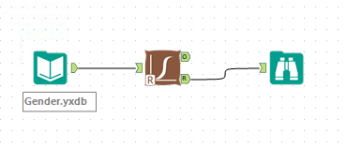We notice that the Logistic Regression tool has one input and two outputs. The input is simply the dataset we want to perform the regression on. The bottom output, the R output, returns a pre fabricated report of the regression when connection a browse tool. The top output, the O output, returns an R object containing the characteristics of the model for further analysis. Note that this R object only can be used with R based tools.

Below the configurations pane of the Logistic Regression tool is displayed.First we need to assign a name to the model.

Secondly we need to select the desired target value, this is our dependent variable gender. Thereafter we select the predictor variables, the variables that we want to predict gender based on, in our case Height and Weight.

Lastly we have to decide the type of logistical regression. Here we have the option of Logit and Probit. The previous are two different means of making the estimation and differs in mathematical properties. In our example the Logit model type is used.

Econometric modelling is a science of its own with plenty of literature explaining the correct methodological approach, this is however beyond the scope of this post. So for now, we will just happily move forward with the choice of the Logit model.

If we inspect the output contained in the pre fabricated report we can inspect the significance of the variables included in the model, as well as their correlations with the response variable, in our case gender.

The model we are developing is quite simple and when considering the significance of the variables, we find that the we have more than desirable levels of significance.Now we have a model containing a ruleset, so to speak, of how to predict gender based on height and weight.

Making predictions

The goal is now to leverage the model in predicting the gender of the individuals in our dataset. This we do through the Score tool which is to be found in the Predictive pane of Alteryx.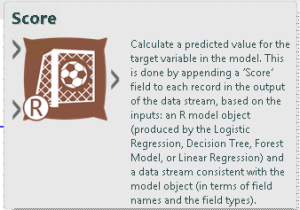The Score tool allows us to assign a probability of being male or female for each observation in our dataset, based on our model.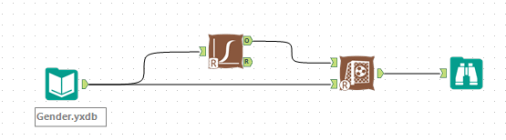In opposition to the Logistic Regression tool, the Score tool contains two inputs and only one output. The top input takes in the R object from a model, in our case the Logistic Regression. The bottom input takes in the dataset on which the predictions are wanted.

In our example we will disregard the configurations pane of the Score tool as we haven't made any modifications to the data, such as oversampling, that we need to make the Score tool aware of.

The output of the Score tool is the inputted dataset with two attached fields. The dataset now also contains the probability of being either male or female.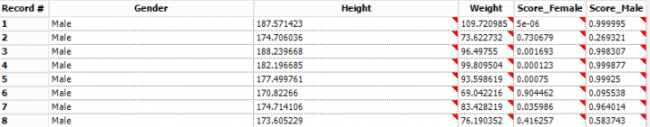Evaluating the model

In the last step of our very basic example we'll evaluate the predictive accuracy of the model. This can be done in many ways, in this post we will use the Lift Chart tool to evaluate the predictive accuracy.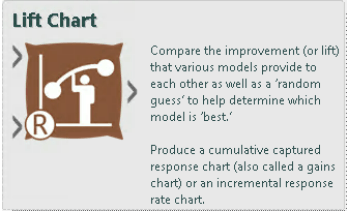The Lift Chart tool is like the other tools used in this post to be found in the Predictive pane of Alteryx. The tool is used to measure the captured response of the predictive model. Let's say we are interested in identifying all the males in our dataset, the lift chart tells us how many of the males in the dataset are identified when looking through different proportions of the dataset.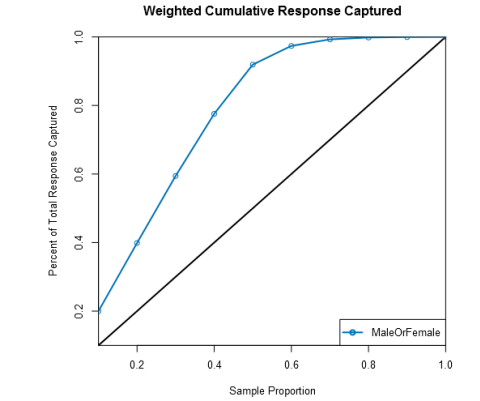Above we see the output of the Lift Chart tool. The Lift Chart should be looked upon as follows:

When looking at 20% of the dataset we’re able of capturing 40% of the males in the data, when looking at 30% of the data our model is able of capturing 60% of the males and so forth.

A neat advantage of the Lift Chart tool is the capability of comparing a variety of models. In that way we're able of identifying the best performing model out of many.

Below the configurations of the Lift Chart tool can be seen.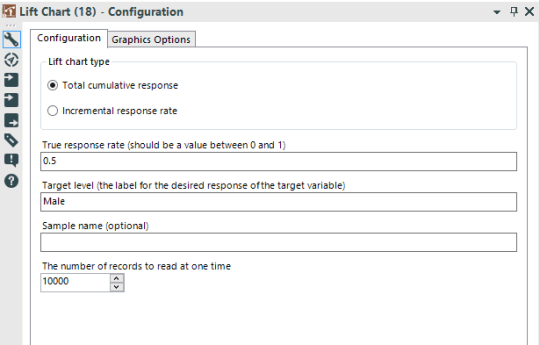Above we simply specify that we want to see a chart of the total cumulative response rate, that the dataset we evaluate contains 50% males and that we are looking for observations of males.

The information that the dataset contains 50% males is a previously done calculation made with the Summarize tool.

Below is the entire workflow can be observed.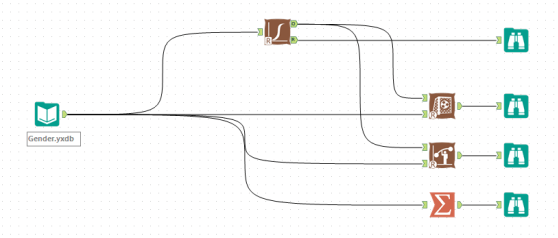In conclusion we now have a way of predicting the gender of an individual with only knowledge of their height and weight. The task is quite neat as there are strong correlations between height, weight and gender. When trying to make predictions on more complicated relationships the complexity of the model will of course increase, however this post present the basic framework of making prediction with Alteryx.

Back to blog